## Design Preloader Totally by ActionScript

The preloader mentioned in this tutorial are totally designed by ActionScript. All of them can be made by copying the ActionScript. I suggest you could read these codes carefully and learn something about ActionScript if you know about AS. Surely you can get more excellent preloader effect in you further creation. One more thing, we don’t offer the source file here.

Series: SWF Quicker 2.0
Estimated Time of Completion: 30 minutes

For that different frame in SWf has different content, the load speed of frames are always different. It is not exact to get the load proportion by _currentframe and _totalframes.

In this tutorial, we use _root.getBytesLoaded () which is the number of bytes loaded in memory and _root.getBytesTotal() which is the total frame number of movie to get the more exact proportion.

1. Add a blank frame in frame 1; the animation begins to play in frame 2.

2. Copy the following codes in frame 1; the movie begins to play as far as it is loaded completely.

Source code here:

 Code: stop ();     _root.createTextField ( "myload_txt" , 1 , 0 , 0 , 0 , 0 ); with ( _root.myload_txt ) { //set the text   background = true ; //whether the text block has a background or not;   backgroundColor = 0x336699 ; //background color of text block;   textColor = 0xFFFFFF ; //text color;   type = "dynamic";//font type is dynamic;   selectable = false ; //whether the text can select or not;   autoSize = "center" ; //control auto sizing and align of text;   _x = Stage.width/2; // x-axis of the text;   _y = Stage.height/2;// y-axis  of the text; } onEnterFrame = function () {   var Loaded = _root.getBytesLoaded ();   var Total = _root.getBytesTotal ();   _root.myload_txt.text = Math.floor (( Loaded / Total )* 100 )+ "%" ;   if ( Loaded == Total ) {     onEnterFrame = null ;     removeMovieClip ( _root.myload_txt );     play ();   } };

1. Designing Truth
The truth for designing preloader can be divided into three parts:
Step one: create a circle to update data. Two ways as follows:
(1) make preloader in two frames and use command gotoAndPlay(1) to generate circle;
(2) Make it in one frame and use command onEnterFrame to generate circle.
Step two: use command get getBytesTotal()and getBytesLoaded() to get file data.
Step three: use graphic or animation to show the date got in step two. Generally, the data can be exactly shown in text.

2. A Simple Sample
I will show you an example step by step:
(1) Import a movie in SWF Quicker and create a new scene put ahead the scene panel.¡¡
(2) Add the following ActionScript in first frame:

 Code: stop (); //four function for drawing lines, they are movie clip name of drawing line, depth of movie clip; //width and transparent of line; function dr ( nam , de , d , al ) {   na = createEmptyMovieClip ( nam , de );   na . lineStyle ( d , 0x9900cc , al );   na . _x = Stage.width/2-120 ;   na . _y = Stage.height/2 ;   na . lineTo ( 240 , 0 ); } onEnterFrame = function () {   var a = getBytesTotal ();   var b = getBytesLoaded ();   //get loaded data;   if ( b < a ) {     dr ( "b1" , 0 , 30 , 30 );     dr ( "b2" , 1 , 20 , 30 );     dr ( "b3" , 2 , 20 , 100 );     b3 . _xscale = b / a * 100 ;   //draw three line to show load progress; } else {     delete onEnterFrame ;     b1 . removeMovieClip ();     b2 . removeMovieClip ();     b3 . removeMovieClip ();     play ();   //load over, delete graphic and function;   } };

Here is final effect of this sample:

 Code: stop(); sw = Stage.width; sh = Stage.height; // Stage.showMenu = false; //hide the context menu of right button; cs = 60; //grid density r = 2550; d = r/Math.SQRT2; //grid slope; p = Math.PI; //customize constant PI createEmptyMovieClip("ln", 1); ln.lineStyle(0, 0xffffff); for (var i = 1; i<=cs; i++) {     ln.moveTo(sw/2+r*Math.cos(i/cs*p), -d+300+r*Math.sin(i/cs*p));     ln.lineTo(sw/2+r*Math.cos(i/cs*p+p/2), -d+300+r*Math.sin(i/cs*p+p/2)); } //draw a background with gradient color; createEmptyMovieClip("bg", 0); with (bg) {     colors = [0x6666ff, 0xffffff, 0x660099];     alphas = [30, 30, 80];     ratios = [0, 100, 200];     lineStyle(5, 0x00ff00);     matrix = {matrixType:"box", x:200, y:115, w:50, h:450, r:p/2};    beginGradientFill("linear", colors, alphas, ratios, matrix);     moveTo(-200-sw, -100);     lineTo(200+sw, -100);     lineTo(200+sw, 100+sh);     lineTo(-200, 100+sh);     lineTo(-200, -100);     endFill(); } // r1 = 50; r2 = 170; nu = 32; //they are three parameters of center circle; cr = 600; //cr is radii of center circle; createTextField("te", 5, 75, 100, 160, 100); te.textColor = 0x9900ff; //create text to show loaded data; function fo1(nam, de, ro) {     na = createEmptyMovieClip(nam, de);     with (na) {         _y = 40;         lineStyle(0, 0x000000, 0);         colors = [0x6666ff, 0xffffff, 0x660099];         alphas = [130, 130, 20];         ratios = [0, 120, 200];         matrix = {matrixType:"box", x:-cr/2, y:-cr/2, w:cr, h:cr, r:p/2};         beginGradientFill("radial", colors, alphas, ratios, matrix);         //Use ActionScript to make gradient fill;         moveTo(r1, 0);         var bl = Math.cos(p/nu);         for (var i = 1; i<=ro; i++) {             curveTo(r1*Math.cos(i*p/(nu/2)-p/nu)/bl, r1*Math.sin(i*p/(nu/2)-p/nu)/bl, r1*Math.cos(i*p/(nu/2)), r1*Math.sin(i*p/(nu/2)));         }         lineTo(r2*Math.cos(ro*p/(nu/2)), r2*Math.sin(ro*p/(nu/2)));         for (var i = ro; i>=1; i--) {             curveTo(r2*Math.cos(i*p/(nu/2)-p/nu)/bl, r2*Math.sin(i*p/(nu/2)-p/nu)/bl, r2*Math.cos((i-1)*p/(nu/2)), r2*Math.sin((i-1)*p/(nu/2)));         }         lineTo(r1, 0);         endFill();     } } //use this function to draw center circle, which is draw by curverTo; onEnterFrame = function () {     ab = _root.getBytesLoaded();     bb = _root.getBytesTotal();     //get data     sb = int(ab/bb*nu);     fo1("di", 4, sb);     di._x = 275;     di._y = 200;     di._yscale = 25;     di._rotation = -30;     //call function to draw     fo1("yz", 3, sb);     yz._xscale = 100*Math.pow(3, 0.5)/2;     yz._yscale = 25;     yz._alpha = 30;     yz._x = 275;     yz._y = 360;     //make another transparent center circle at the bottom as shadow of upper one;    if (ab>>>;"       di.onPress = function() {          delete onEnterFrame;          te.removeTextField();          di.removeMovieClip();          yz.removeMovieClip();          ln.removeMovieClip();          bg.removeMovieClip();          play();         };     } };

That is the final effect:

 Code: onClipEvent (load) {    this._x = 180;    this._y = 300;    _root.ss.createTextField("tt", 1, 70, 50, 100, 20);    _root.ss.tt.textColor = 0xff0000;    _root.ss.createEmptyMovieClip("louding", 2);    with (_root.ss.louding) {       lineStyle(0, 0x0000ff, 0);       moveTo(0, 0);       //originating point;       beginFill(0xff0000, 100);       lineTo(0, 10);       lineTo(10, 10);       lineTo(10, 0);       endFill();    }    _root.ss.createEmptyMovieClip("loudingk", 3);    with (_root.ss.loudingk) {       lineStyle(0, 0x000000, 100);       moveTo(0, 0);       //originating point;       lineTo(0, 10);       lineTo(200, 10);       lineTo(200, 0);       lineTo(0, 0);    } } onClipEvent (enterFrame) {    load = int(_root.getBytesLoaded()/_root.getBytesTotal()*100);    _root.ss.tt.text = "loading"+load+"%";    _root.ss.louding._width = 2*load;    if (_root.getBytesLoaded() == _root.getBytesTotal()) {       _root.play();    } }

That is the effect: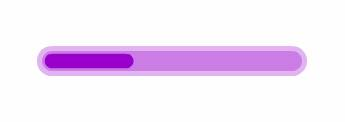1. Import a movie in SWF Quicker and add a new scene ahead of Scene panel.
2. Add the following ActionScript in the first frame of the new scene.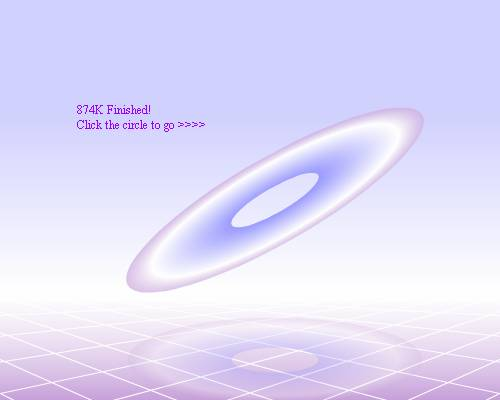1. Open a movie in SWF Quicker and add a new scene ahead of Scene panel.
2. Create a new movie clip and drag it to first frame of new scene, name it "ss" in properties panel.
3. Add the following ActionScript in the first frame of the new scene.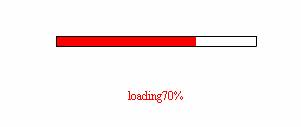Sothink is a trademark of SourceTec Software Co., LTD.•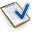Support
•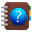Tutorials
•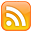Subscribe
•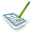Blog
•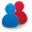Forum
•Affiliate